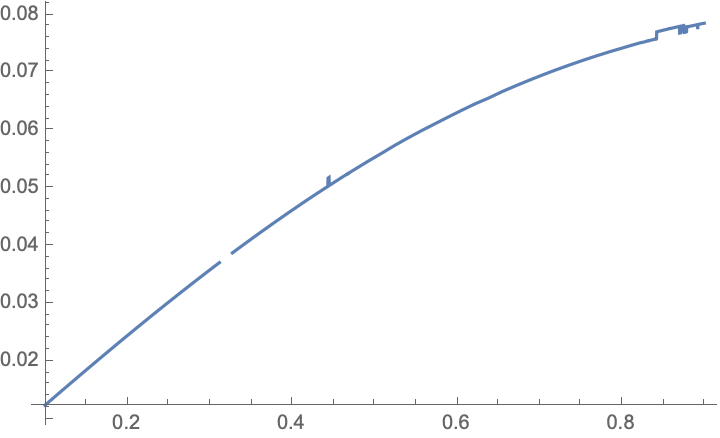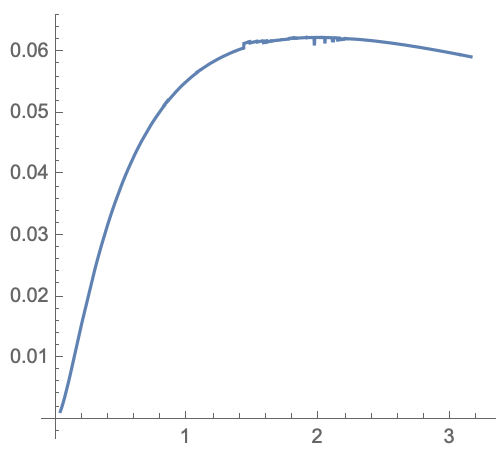# distribution on the inverse Wishart matrix eigenvalues summation

Let $$\lambda_1>\lambda_2>....>\lambda_N$$ be the ordered eigenvalues of Wishart matrix my objective is to find if it is possible the distribution of:

\begin{align} s = \sum\limits_{i = 1}^N {\frac{1}{{1 + a{\lambda _i}}}} \end{align} where a is positive and $$N\ge 2$$.

For small $$N$$ explicit expressions are cumbersome. For $$N\gg 1$$ the distribution $$P(s)$$ is a Gaussian. The mean is given by integration with the Marchenko-Pastur distribution, the variance is given by integration with a formula given in arXiv:9310010, Equation 17. Let me work this out:

The Wishart matrix is $$X=WW^T$$, with $$W$$ an $$N\times M$$ real matrix, $$N\leq M$$ and $$y=N/M$$. I rescale the eigenvalues $$\lambda_i$$ of $$X$$ by $$x_i=\lambda_i/M$$, and define $$\alpha=aM$$. We seek the distribution of $$s=\sum_{i}(1+\alpha x_i)^{-1}$$. The support of the eigenvalue density $$\rho(x)$$ is the interval $$(a_-,a_+)$$, with $$a_\pm=(1\pm\sqrt y)^2$$. For $$N\gg 1$$ one has the Marchenko-Pastur distribution $$\rho(x)=\frac{1}{2\pi}\frac{N}{yx}(x-a_-)^{1/2}(a_+-x)^{1/2},$$ normalized to $$\int\rho(x)dx=N$$. The mean of the Gaussian is then equal to $$\mathbb{E}[s]=\int_{a_-}^{a_+}\frac{\rho(x)}{1+\alpha x}\,dx=N\frac{\sqrt{\alpha^2 (y-1)^2+2 \alpha (y+1)+1}+\alpha (y-1)-1}{2 \alpha y}.$$

For the variance we apply Eq. 17 of the cited paper, $${\rm var}\,s=\frac{1}{\pi^2}\int_{\alpha_-}^{a_+}d\lambda\int_{a_-}^{a_+}d\mu\frac{\sqrt{(\mu-a_-)(a_+-\mu)}}{\sqrt{(\lambda-a_-)(a_+-\lambda)}}\frac{1}{\lambda-\mu}\frac{1}{1+\alpha\lambda}\frac{d}{d\mu}\frac{1}{1+\alpha\mu},$$ the integrals being Cauchy principal values.
I have not succeeded in evaluating the integrals in closed form. Here is a numerical calculation of the variance for $$\alpha=1$$ as a function of $$y$$,and a plot of the variance for $$y=0.5$$ as a function of $$\alpha$$,For the numerical evaluation it is convenient to rewrite the integral in the form $${\rm var}\,s=\frac{1}{\pi^2}\int_{a_-}^{a_+}d\lambda\int_{a_-}^{a_+}d\mu\ln|\lambda-\mu|\frac{d}{d\mu}\left(\frac{\sqrt{(\mu-a_-)(a_+-\mu)}}{\sqrt{(\lambda-a_-)(a_+-\lambda)}}\frac{1}{1+\alpha\lambda}\frac{d}{d\mu}\frac{1}{1+\alpha\mu}\right).$$